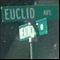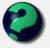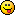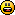Discussion about math, puzzles, games and fun.   Useful symbols: ÷ × ½ √ ∞ ≠ ≤ ≥ ≈ ⇒ ± ∈ Δ θ ∴ ∑ ∫  π  -¹ ² ³ °

You are not logged in.

## #1 2006-03-18 11:43:30

Ricky
ModeratorRegistered: 2005-12-04
Posts: 3,791

### Euler's forumula

It is a pretty cool thing:

One professor of mine made a pretty bold claim the other day about it.  He said that any trig identity can be derived using this and only this formula.  So here's an idea.  Take a trig identity and see if you can prove it using nothing else.  If you can, post it here.  Any identity that we can prove using only Euler's Formula is fair game (i.e. you can use it in another proof), but you gotta prove it first before you can use it.

If you run into one that you have trouble with, by all means, post it.

I'm a bit short on time, so I'll post one up later tonight.

Edit:

Whoops, thanks mathsyperson

Last edited by Ricky (2006-03-19 04:24:09)

"In the real world, this would be a problem.  But in mathematics, we can just define a place where this problem doesn't exist.  So we'll go ahead and do that now..."

Offline

## #2 2006-03-19 02:08:57

mathsyperson
ModeratorRegistered: 2005-06-22
Posts: 4,900

### Re: Euler's forumula

Should that be

?

I'm not that good with complex numbers, so I'm not sure how useful I'll be, but I'll give it a go later on, when I've got some more free time.

Why did the vector cross the road?
It wanted to be normal.

Offline

## #3 2006-03-19 04:23:47

Ricky
ModeratorRegistered: 2005-12-04
Posts: 3,791

### Re: Euler's forumula

The only real property of imaginary numbers you really have to know is that:

i * i = -1
if a + bi = c + di, then a = c and b = d.

That said, here's cos(2θ) = cos²θ - sin²θ:

"In the real world, this would be a problem.  But in mathematics, we can just define a place where this problem doesn't exist.  So we'll go ahead and do that now..."

Offline

## #4 2006-03-19 04:26:49

Ricky
ModeratorRegistered: 2005-12-04
Posts: 3,791

### Re: Euler's forumula

There is another property that is hidden in there if you can find it.  Remember:

if a + bi = c + di, then a = c and b = d.

I used the real parts. Someone can still use the imaginary.

"In the real world, this would be a problem.  But in mathematics, we can just define a place where this problem doesn't exist.  So we'll go ahead and do that now..."

Offline

## #5 2006-03-19 04:51:01

mathsyperson
ModeratorRegistered: 2005-06-22
Posts: 4,900

### Re: Euler's forumula

Yes, I noticed that. OK, I'll shamelessly steal your LaTeX and alter it for my own purposes then.Why did the vector cross the road?
It wanted to be normal.

Offline

## #6 2006-03-19 05:08:16

Ricky
ModeratorRegistered: 2005-12-04
Posts: 3,791

### Re: Euler's forumula

Nicely done.  Now I suggest we go after cos²θ + sin²θ = 1, as that will most likely be a useful property to use for other identites later on.

If no one gets it by tomorrow, I'll post a hint.

"In the real world, this would be a problem.  But in mathematics, we can just define a place where this problem doesn't exist.  So we'll go ahead and do that now..."

Offline

## #7 2006-03-19 14:56:48

George,Y
MemberRegistered: 2006-03-12
Posts: 1,372

### Re: Euler's forumula

e^ix e^-ix = (cosx+isinx)(cosx-isinx) < sin(-x) =-sinx > = (cos^2[x] - i^2 sin^2[x])=(cos[x])^2+(sin[x])^2

where e^ix e^-ix =1

X'(y-Xβ)=0

Offline

## #8 2006-03-19 15:57:25

ganeshRegistered: 2005-06-28
Posts: 30,317

### Re: Euler's forumula

Absolutely right, George Y.

.
By Abraham de Moivre's theorem,

(Cosx+iSinx)(Cosx-iSinx)=Cos²x - (i²)Sin²x = Cos²x - (-1)Sin²x
= Cos²x + Sin²x

Therefore, Cos²x + Sin²x=1.

It is no good to try to stop knowledge from going forward. Ignorance is never better than knowledge - Enrico Fermi.

Nothing is better than reading and gaining more and more knowledge - Stephen William Hawking.

Offline

## #9 2006-03-19 16:32:43

Ricky
ModeratorRegistered: 2005-12-04
Posts: 3,791

### Re: Euler's forumula

One property used in that proof is cos(x) = cos(-x) and sin(x) = -sin(x).  I have yet to find a way to prove these using e^iθ, but for the moment, do so using the definitions of sine and cosine.

Last edited by Ricky (2006-03-19 16:47:25)

"In the real world, this would be a problem.  But in mathematics, we can just define a place where this problem doesn't exist.  So we'll go ahead and do that now..."

Offline

## #10 2006-03-21 13:22:10

Ricky
ModeratorRegistered: 2005-12-04
Posts: 3,791

### Re: Euler's forumula

Actually, let's skip cos(x) = cos(-x) and sin(x) = -sin(-x) for now.  I believe they will come up soon enough.

Prove that cos(a+b) = cos(a)cos(b) - sin(a)sin(b).

"In the real world, this would be a problem.  But in mathematics, we can just define a place where this problem doesn't exist.  So we'll go ahead and do that now..."

Offline

## #11 2006-03-23 03:21:31

George,Y
MemberRegistered: 2006-03-12
Posts: 1,372

### Re: Euler's forumula

Circular proof, i am sorry to say

Why don't you explore other applications, to which cannot be solved simply within trigonometric formulas?

eg.

Sin[A] Sin[b] Sin[C]

X'(y-Xβ)=0

Offline

## #12 2006-03-23 03:22:44

George,Y
MemberRegistered: 2006-03-12
Posts: 1,372

### Re: Euler's forumula

Though a very good angle to memorize those formulasX'(y-Xβ)=0

Offline

## #13 2006-03-23 09:13:19

Ricky
ModeratorRegistered: 2005-12-04
Posts: 3,791

### Re: Euler's forumula

And why exactly is it circular, George?

"In the real world, this would be a problem.  But in mathematics, we can just define a place where this problem doesn't exist.  So we'll go ahead and do that now..."

Offline

## #14 2006-03-23 13:31:53

George,Y
MemberRegistered: 2006-03-12
Posts: 1,372

### Re: Euler's forumula

because in order to prove Euler's formula, we commonly use Taylor series.

And both Sine and Cosine Taylor series are derived via making derivatives (i don't know how to use a single verb to say it) of themselves.

Sine's derivative's proof has an essential part:

Sin(x+Δx) - Sin(x) = 2Sin((x+Δx-x)/2)Cos((x+Δx+x)/2)=2Sin(Δx/2)Cos(x+Δx/2)

More or less does cosine'sLast edited by George,Y (2006-03-23 13:33:46)

X'(y-Xβ)=0

Offline

## #15 2006-03-23 16:40:49

Ricky
ModeratorRegistered: 2005-12-04
Posts: 3,791

### Re: Euler's forumula

George, I agree.  And actually, I agreed before you ever backed up your statement.  I really just wanted you to start showing what you said instead of just stating it.But I think you missed the entire point of this topic.  There is only one trig formula, and if you accept this formula, then you can generate every single other trig identity.

We aren't proving things.  Now I have used the word prove before, but what I meant was "assuming that e^iθ = cosθ + isinθ, prove that ...."  Sorry if the wording I used was bad, I should have used "show" instead of "prove."

"In the real world, this would be a problem.  But in mathematics, we can just define a place where this problem doesn't exist.  So we'll go ahead and do that now..."

Offline

## #16 2006-03-24 01:21:01

George,Y
MemberRegistered: 2006-03-12
Posts: 1,372

### Re: Euler's forumula

Never mind. we just have different anglesX'(y-Xβ)=0

Offline

## #17 2006-04-07 18:09:44

George,Y
MemberRegistered: 2006-03-12
Posts: 1,372

### Re: Euler's forumula

It really has an great application!!
Beyond simple trigonometric formulas

It can be used to derive cos(nθ) and sin(nθ) by cos(θ) and sin(θ).

...

last part ,+ -uncertain,

when n is even,

or

when n is oddLast edited by George,Y (2006-04-07 18:55:10)

X'(y-Xβ)=0

Offline

## #18 2006-04-09 04:24:17

George,Y
MemberRegistered: 2006-03-12
Posts: 1,372

### Re: Euler's forumula

cos(180°)=
cos(45° )[sup]4[/sup]-6cos(45° )[sup]2[/sup]sin(45°)²+sin(45°)[sup]4[/sup]
=1/4-6(1/4)+1/4=-1

0=cos(90°)=cos[sup]5[/sup](18°) -10cos³(18°)sin²(18°)+5cos(18°)sin[sup]4[/sup](18°)

define p=cos²(18°) hence sin²(18°)=1-p

p²-10p(1-p)+(1-p)²=0

X'(y-Xβ)=0

Offline

## #19 2006-04-09 13:05:15

George,Y
MemberRegistered: 2006-03-12
Posts: 1,372

### Re: Euler's forumula

p²-10p(1-p)+5(1-p)²=0 corrected
16p²-20p+5=0

p= (20±√80)/32 = (5+√5)/8 = (10+2√5)/16

1-p= (3-√5)/8

cos(18°) = √p or=+√p

since no one can solve that out

i just compute  cos(36°) = 2p-1 = (1+√5)/4 , cos²(36°)= 1-previous²
and

Last edited by George,Y (2006-04-09 13:06:15)

X'(y-Xβ)=0

Offline